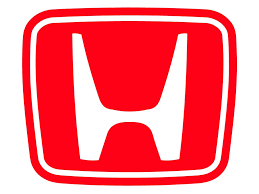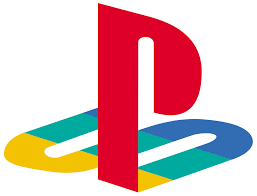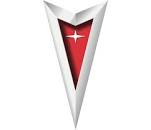Movies
Technology
Car Companies
Angles
Lines, Planes
100The God Father

100

What does this logo stand for ?Apple

100

What does this logo stand for ?Honda

100

The sum of the angles are 90.

Complementary Angles

100

The intersection of two lines makes ......

Point

200The Black Panther

200

What does this logo stand for ?200

What does this logo stand for ?Toyota

200

The perpendicular lines make an angle of

900

200

The intersection of planes makes a .............

line

300Ex Machina

300

What does this logo stand for ?Windows

300

What does this logo stand for ?Mitsubishi

300

The vertical angles are always ....................

Congruent (equal)

300

How many points are needed to define a plane?

3

400Coco

400

What does this logo stand for ?X Box

400

What does this logo stand for ?Mercedes-Benz

400

Angles x and 2x are linear pair. What is the smallest angle?

600

400

The midpoint of (2,5) and (4,-1) is

(3,2)

500Gravity

500

What does this logo stand for ?Playstation

500

What does this logo stand for ?Pontiac

500

The angles x and 2x-10 are congruent. What is the smallest angle?

100

500

If the line AB=x and the line BC=2x-5 and B is the midpoint so what is x?

5

Click to zoom